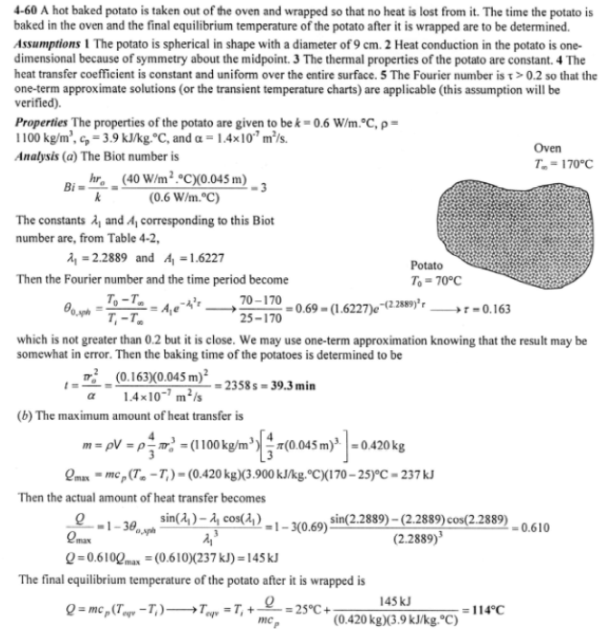heat and mass transfer problems engineering equations heat and mass transfer school homework engineering heat and mass transfer formulas heat and mass transfer solutions to heat and mass transfer problems full solution engineering problem solution heat and mass transfer math problems engineering equations heat and mass transfer school homework engineering solutions to heat and mass transfer formulas heat problems mass problem solutions to transfer problems full solution heat and mass transfer
heat and mass transfer problems engineering equations heat and mass transfer school homework engineering heat and mass transfer formulas heat and mass transfer solutions to heat and mass transfer problems full solution engineering problem solution heat and mass transfer math problems engineering equations heat and mass transfer school homework engineering solutions to heat and mass transfer formulas heat problems mass problem solutions to transfer problems full solution heat and mass transfer
Highalphabet Home Page heat and mass transfer problem solutions Heat and Mass Transfer Page
A 9-cm-diameter potato (rho=1100 kg/m^3, c_p=3900 J/kgK, k=0.6 W/mK, and alpha=1.4x10^-7 m^2/s) that is initially at a uniform temperature of 25 C is baked in an oven at 170 C until a temperature sensor inserted to the center of the potato indicates a reading of 70 C. The potato is then taken out of the oven and wrapped in thick towels so that almost no heat is lost from the baked potato. Assuming the heat transfer coefficient in the oven to be 40 W/m^2 K, determine (a) how long the potato is baked in the oven and (b) the final equilibrium temperature of the potato after it is wrapped.A 9-cm-diameter potato (rho=1100 kg/m^3, c_p=3900 J/kgK, k=0.6 W/mK, and alpha=1.4x10^-7 m^2/s) that is initially at a uniform temperature of 25 C is baked in an oven at 170 C until a temperature sensor inserted to the center of the potato indicates a reading of 70 C. The potato is then taken out of the oven and wrapped in thick towels so that almost no heat is lost from the baked potato. Assuming the heat transfer coefficient in the oven to be 40 W/m^2 K, determine (a) how long the potato is baked in the oven and (b) the final equilibrium temperature of the potato after it is wrapped.